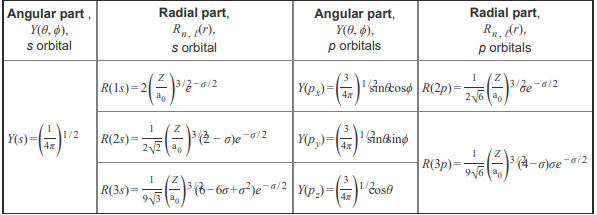# Problem: Part A. The s orbital is spherical (nondirectional) because  Check all that apply.a) the angular wave function for all s orbitals is a constant. b) at a particular distance from the nucleus, the electron probability is the same in all directions. c) the angular wave function of an s orbital is defined by the quantum number ℓ. d) an s orbital has angular nodes. e) the angular wave function of an s orbital varies with the principal quantum number (n).The atomic orbitals are wave functions (ψ) that are mathematical solutions of the Schrödinger wave equation, and the atomic orbitals are represented mathematically by ψ(r, θ, ϕ) = R(r)Y(θ, ϕ)The function R(r) is called the radial wave function, where r is the distance of the electron from the nucleus of an atom where the probability of finding an electron is at a maximum, and the function Y(θ, ϕ) is called the angular wave function, where θ and ϕ represent different angles describing the orientation of the distance line, r , with respect to the x, y, and z axes. The electron probability density is expressed in terms of ψ2 . It is the probability (per unit volume) of finding the electron at a point in space. The region where electron probability density is zero is called a node. A node may be an angular node or a radial node. The solutions to the Schrödinger wave equation are characterized by three quantum numbers: n,ℓ, and mℓ . 1. The principal quantum number n determines the size and energy of the orbital. 2. The orbital angular momentum (or azimuthal) quantum number ℓ determines the angular distribution or the shape of an orbital. 3. The magnetic quantum number mℓ determines the orientation of the orbital. Angular and radial wave functions for s and p orbitalsThe equations for the angular and radial wave functions for the s and p orbital may be useful when completing this tutorial.

###### FREE Expert Solution
81% (302 ratings)View Complete Written Solution
###### Problem Details

Part A. The s orbital is spherical (nondirectional) because

Check all that apply.

a) the angular wave function for all s orbitals is a constant.

b) at a particular distance from the nucleus, the electron probability is the same in all directions.

c) the angular wave function of an s orbital is defined by the quantum number ℓ.

d) an s orbital has angular nodes.

e) the angular wave function of an s orbital varies with the principal quantum number (n).

The atomic orbitals are wave functions (ψ) that are mathematical solutions of the Schrödinger wave equation, and the atomic orbitals are represented mathematically by

ψ(r, θ, ϕ) = R(r)Y(θ, ϕ)

The function R(r) is called the radial wave function, where r is the distance of the electron from the nucleus of an atom where the probability of finding an electron is at a maximum, and the function Y(θ, ϕ) is called the angular wave function, where θ and ϕ represent different angles describing the orientation of the distance line, r , with respect to the x, y, and z axes.

The electron probability density is expressed in terms of ψ2 . It is the probability (per unit volume) of finding the electron at a point in space. The region where electron probability density is zero is called a node. A node may be an angular node or a radial node.

The solutions to the Schrödinger wave equation are characterized by three quantum numbers: n,, and m

1. The principal quantum number n determines the size and energy of the orbital.

2. The orbital angular momentum (or azimuthal) quantum number ℓ determines the angular distribution or the shape of an orbital.

3. The magnetic quantum number mℓ determines the orientation of the orbital.

Angular and radial wave functions for s and p orbitals

The equations for the angular and radial wave functions for the s and p orbital may be useful when completing this tutorial.